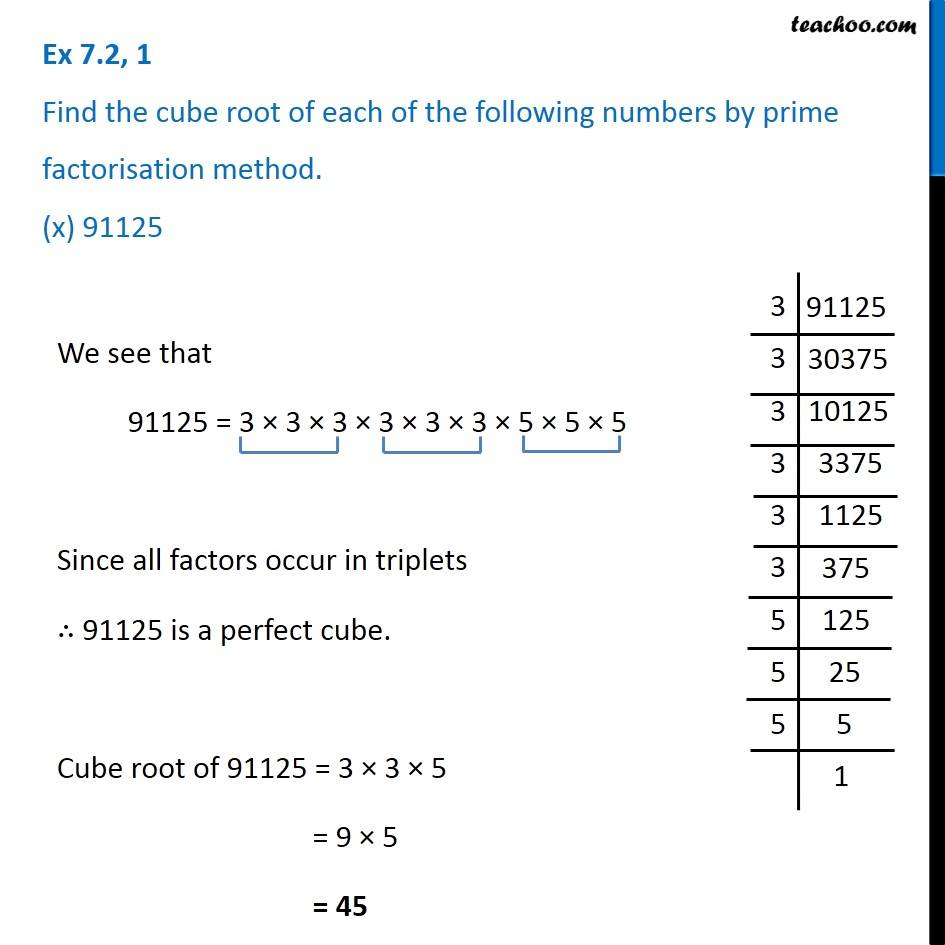Ex 7.2

Chapter 7 Class 8 Cubes and Cube Roots
Serial order wiseIntroducing your new favourite teacher - Teachoo Black, at only ₹83 per month

### Transcript

Ex 7.2, 1 Find the cube root of each of the following numbers by prime factorisation method. (x) 91125We see that 91125 = 3 × 3 × 3 × 3 × 3 × 3 × 5 × 5 × 5 Since all factors occur in triplets ∴ 91125 is a perfect cube. Cube root of 91125 = 3 × 3 × 5 = 9 × 5 = 45